# Notebook for 'Measuring and controlling knowledge diversity'¶

Jérôme Euzenat, Yasser Bourahla, 08/2022

This notebook contains code and results for the paper 'Measuring and controlling diversity'.

If you see this notebook as a simple HTML page, then it has been generated by the notebook found in this archive.

This is not a maintained software package, technically just a notebook. But if you want to use it, feel free to do it under the MIT License.

## Ontology distributions¶

Here are the 7 distributions of the paper (a,b,c,d,e,f,g) of 5 ontologies (A, B, C, D, E) among 10 agents. They are encoded as arrays.

We provide three extra distributions (h, i, j), for the sake of trying.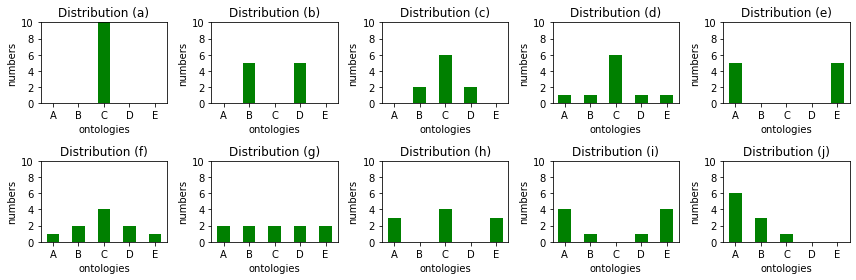## Distances¶

The distances between the 5 ontologies are coded into arrays.

So there are no program connection between knowledge distance and diversity.

Such measures may be found in:

unstructdist
A B C D E
A 0 1 1 1 1
B 1 0 1 1 1
C 1 1 0 1 1
D 1 1 1 0 1
E 1 1 1 1 0

linearstructdist
A B C D E
A 0 1 2 3 4
B 1 0 1 2 3
C 2 1 0 1 2
D 3 2 1 0 1
E 4 3 2 1 0

The initial distances from the submitted version have been changed

There has been two changes:

(1) the order in the matrix was the current CBDEA

(2) A (E at submission) was actually different --affecting only the second

graphsemdist
A B C D E
A 0.00 0.33 0.67 1.00 1.00
B 0.33 0.00 0.33 1.00 1.00
C 0.67 0.33 0.00 0.33 0.67
D 1.00 1.00 0.33 0.00 0.33
E 1.00 1.00 0.67 0.33 0.00

namesemdist
A B C D E
A 0.00 0.43 0.71 0.57 0.29
B 0.43 0.00 0.50 0.50 0.67
C 0.71 0.50 0.00 0.50 0.67
D 0.57 0.50 0.50 0.00 0.33
E 0.29 0.67 0.67 0.33 0.00

## Diversity measures¶

The code for computing various diversity measures is provided here. It could be made a separate Python library if needed, but this is not yet the case.

They implement a signature: diversity( distrib, dissimilarity ): float

These are:

• structdist: computes the average distance between the categories of the distribution;
• calcdiam: computes the diameter of the distribution;
• median: computes the median of the distribution.

The entropy-based diversity measures are provided into two favours:

• entropy (additional parameter q): compute the generalised entropy-based diversity measure. This is the initial naïve version;
• diversity (additional parameter q): a better implemented version of diversity-based entropy which also includes the implementation of the limit case $q=1$.

The normalised versions are included but must be used with care as they are only correct if the maximal value is given by equi-distributed distributions (which is not necessary the case).

## Results¶

Finally the results to be found in Table 2 of the paper are gathered here.

These results include, in addition of those submitted:

• results for the median (now published, with standard deviation not published),
• results with the new ontology A,
• distribution (e) has become (h), distribution (b) has become (e), a new distribution (b) is introduced,
• results with the additional distributions (h-i-j).
a b c d e f g h i j
categ A 0.00 0.00 0.00 1.00 5.00 1.00 2.00 3.00 4.00 6.00
B 0.00 5.00 2.00 1.00 0.00 2.00 2.00 0.00 1.00 3.00
C 10.00 0.00 6.00 6.00 0.00 4.00 2.00 4.00 0.00 1.00
D 0.00 5.00 2.00 1.00 0.00 2.00 2.00 0.00 1.00 0.00
E 0.00 0.00 0.00 1.00 5.00 1.00 2.00 3.00 4.00 0.00
stats |A| 10.00 10.00 10.00 10.00 10.00 10.00 10.00 10.00 10.00 10.00
|O| 1.00 2.00 3.00 5.00 2.00 5.00 5.00 3.00 4.00 3.00
|O|/|A| 0.10 0.20 0.30 0.50 0.20 0.50 0.50 0.30 0.40 0.30
nostruct diam 0.00 1.00 1.00 1.00 1.00 1.00 1.00 1.00 1.00 1.00
med 0.00 1.00 1.00 1.00 1.00 1.00 1.00 1.00 1.00 1.00
dist 0.00 0.56 0.62 0.67 0.56 0.82 0.89 0.73 0.73 0.60
stdev 0.00 0.50 0.50 0.49 0.50 0.45 0.41 0.48 0.48 0.50
entr 0.00 0.45 0.54 0.60 0.45 0.86 1.00 0.70 0.70 0.51
linear diam 0.00 2.00 2.00 4.00 4.00 4.00 4.00 4.00 4.00 2.00
med 0.00 2.00 1.00 1.00 4.00 1.00 1.00 2.00 1.50 1.00
dist 0.00 1.11 0.71 1.11 2.22 1.33 1.78 1.87 2.18 0.73
stdev 0.00 1.01 0.63 1.01 2.01 0.99 1.21 1.42 1.73 0.69
entr 0.00 0.43 0.33 0.48 0.54 0.70 1.00 0.81 0.79 0.33
graphsem diam 0.00 1.00 1.00 1.00 1.00 1.00 1.00 1.00 1.00 0.67
med 0.00 1.00 0.33 0.33 1.00 0.33 0.33 0.67 0.67 0.33
dist 0.00 0.56 0.27 0.37 0.56 0.47 0.59 0.56 0.61 0.24
stdev 0.00 0.50 0.28 0.33 0.50 0.35 0.38 0.38 0.46 0.23
entr 0.00 0.78 0.39 0.56 0.78 0.74 1.00 0.90 0.96 0.37
namesem diam 0.00 0.50 0.50 0.71 0.29 0.71 0.71 0.71 0.67 0.71
med 0.00 0.50 0.50 0.50 0.29 0.50 0.50 0.29 0.29 0.43
dist 0.00 0.28 0.31 0.38 0.16 0.44 0.46 0.43 0.29 0.30
stdev 0.00 0.25 0.25 0.30 0.14 0.26 0.24 0.31 0.22 0.27
entr 0.00 0.52 0.61 0.74 0.30 0.94 1.00 0.85 0.57 0.57

## Tentative partial order based on entropic diversity measures¶

Here is a tentative to induce a partial order from the order of diversity.

The algorithm is quite simple:

• Compute the matrix corresponding to distribution x q for values of $q$ ranging among -200 -100 -10 -1 0 .9 1.1 2 10 100 200 (beyond 200 it is too large)
• Compute the matrix distribution x distribution such that it corresponds to the diversity order:
• = all values are equal
• < they are not always equal, some may be superior
• > they are not always equal, some may be inferior
• . sometimes they are inferior, sometimes they are superior

Note: Tom Leinster mentions that he restricts this to $q\geq 0$ (for reasons he does not explain, but which are discussed on page 121 of his book).
The result is as follows:

With unstructured distance

a b c d e f g h i j
a = > > > > > > > > >
b < = . . = > > > > .
c < . = > . > > > > .
d < . < = . > > . > .
e < = . . = > > > > .
f < < < < < = > < < <
g < < < < < < = < < <
h < < < . < > > = . <
i < < < < < > > . = <
j < . . . . > > > > =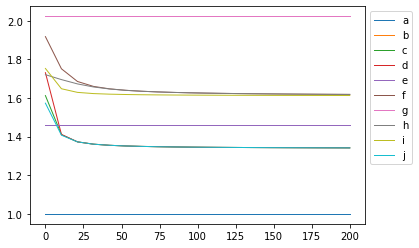With linearly structured distance

a b c d e f g h i j
a = > > > > > > > > >
b < = < . > . > > > <
c < > = > > > > > > .
d < . < = . > > > > <
e < < < . = . > > > <
f < . < < . = > > > <
g < < < < < < = < < <
h < < < < < < > = . <
i < < < < < < > . = <
j < > . > > > > > > =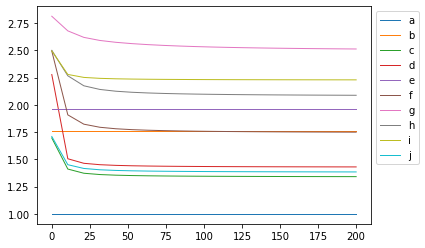With graph-based semantic distance

a b c d e f g h i j
a = > > > > > > > > >
b < = < < = . . . > <
c < > = > > > > > > .
d < > < = > > > > > <
e < = < < = . . . > <
f < . < < . = > > > <
g < . < < . < = < . <
h < . < < . < > = > <
i < < < < < < . < = <
j < > . > > > > > > =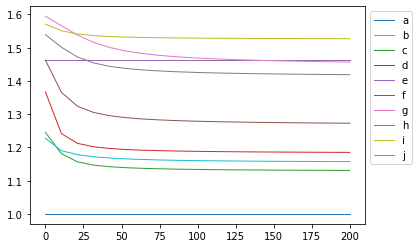With named-class-based semantic distance

a b c d e f g h i j
a = > > > > > > > > >
b < = . . < > > > . .
c < . = > < > > > . <
d < . < = < > > > < <
e < > > > = > > > > >
f < < < < < = > . < <
g < < < < < < = < < <
h < < < < < . > = < <
i < . . > < > > > = .
j < . > > < > > > . =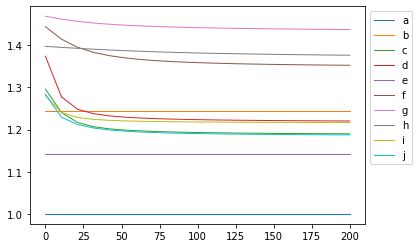## Tentative algorithm for diversity control¶

We start with a distribution and generate distributions with lower diversity. Ideally, it should be possible to start with a high diversity distribution. Then we want to achieve some levels of diversity. This is always with respect to a specific diversity measure.

For that purpose, the algorithm modifies the distribution one agent at a time. It does it so that the diversity decreases minimally at each stage (this is local).

It can be called by selectdistribs( [2,2,2,2,2], unstructdist, 4 ) which will provide a sequence of 4 distributions evenly spread (from the standpoint of the diversity of the non structured distance and $q=2$), from the [2,2,2,2,2] distribution.

It returns the distributions and their (non normalised) diversity level.

The result is:

What is in the paper (Figure 4):

unstrucdist-3
distribution diversity
0 [1, 1, 1] 1.00
1 [2, 0, 1] 0.67
2 [3, 0, 0] 0.00

unstrucdist-4
distribution diversity
0 [1, 1, 1, 1] 1.00
1 [2, 0, 1, 1] 0.83
2 [2, 0, 2, 0] 0.67
3 [3, 0, 1, 0] 0.50
4 [4, 0, 0, 0] 0.00

unstrucdist-5
distribution diversity
0 [1, 1, 1, 1, 1] 1.00
1 [2, 0, 1, 1, 1] 0.90
2 [2, 0, 2, 0, 1] 0.80
3 [3, 0, 1, 0, 1] 0.70
4 [3, 0, 2, 0, 0] 0.60
5 [4, 0, 1, 0, 0] 0.40
6 [5, 0, 0, 0, 0] 0.00

What is in the paper (Figure 5):

More interesting:

unstructdist
distribution diversity
0 [2, 2, 2, 2, 2] 1.00
1 [4, 0, 2, 0, 4] 0.66
2 [3, 0, 0, 0, 7] 0.35
3 [0, 0, 0, 0, 10] 0.00

linearstructdist
distribution diversity
[2, 2, 2, 2, 2] 1.00
[1, 4, 0, 0, 5] 0.63
[2, 7, 0, 0, 1] 0.30
[0, 10, 0, 0, 0] 0.00

graphsemdist
distribution diversity
[2, 2, 2, 2, 2] 1.00
[6, 0, 3, 0, 1] 0.66
[8, 0, 2, 0, 0] 0.31
[10, 0, 0, 0, 0] 0.00

namesemdist
distribution diversity
[2, 2, 2, 2, 2] 1.00
[6, 2, 2, 0, 0] 0.67
[7, 3, 0, 0, 0] 0.37
[10, 0, 0, 0, 0] 0.00

Something interesting in these modest results:

• depending on the diversity measures, different distributions are obtained;
• even the least diverse distribution may be different.